International
Tables for
Crystallography
Volume D
Physical properties of crystals
Edited by A. Authier

International Tables for Crystallography (2013). Vol. D, ch. 1.11, p. 274

## Section 1.11.4. Physical mechanisms for the anisotropy of atomic X-ray susceptibility

V. E. Dmitrienko,a* A. Kirfelb and E. N. Ovchinnikovac

aA. V. Shubnikov Institute of Crystallography, Leninsky pr. 59, Moscow 119333, Russia,bSteinmann Institut der Universität Bonn, Poppelsdorfer Schloss, Bonn, D-53115, Germany, and cFaculty of Physics, M. V. Lomonosov Moscow State University, Leninskie Gory, Moscow 119991, Russia
Correspondence e-mail:  dmitrien@crys.ras.ru

### 1.11.4. Physical mechanisms for the anisotropy of atomic X-ray susceptibility

| top | pdf |

Conventional non-resonant Thomson scattering in condensed matter is the result of the interaction of the electric field of the electromagnetic wave with the charged electron subsystem. However, there are also other mechanisms of interaction, e.g. interaction of electromagnetic waves with spin and orbital moments, which was first considered by Platzman & Tzoar (1970) for molecules and solids. They predicted the sensitivity of X-ray diffraction to a magnetic structure of a crystal, as later observed in the pioneering works of de Bergevin & Brunel (de Bergevin & Brunel, 1972, 1981; Brunel & de Bergevin, 1981). It is reasonable to describe all X-ray–electron interactions by the Pauli equation (Berestetskii et al., 1982), which is a low-energy approximation to the Dirac equation (typical X-ray energies are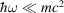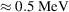where m is the electron mass). The equation accounts for charge and spin interaction with the electromagnetic field of the wave, and spin–orbit interaction (Blume, 1985, 1994) using the following Hamiltonian: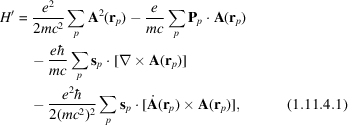where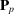is the momentum of the pth electron, and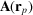is the vector potential of the electromagnetic wave with wavevectorand polarization.

Here and below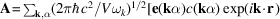+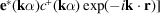, whereis a quantization volume, indexlabels two polarizations of each wave,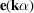are the polarizations vectors, and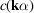and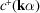are the photon annihilation and creation operators.

Considering X-ray scattering by different atoms in solids as independent processes [in Section 1.2.4of International Tables for Crystallography Volume B, this is called `the isolated-atom approximation in X-ray diffraction'; the validity of this approximation has been discussed by Kolpakov et al. (1978)], the atomic scattering amplitude, which describes the scattering of a wave with wavevectorand polarizationinto a wave with wavevectorand polarization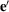, can be written as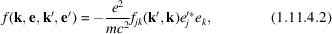where the tensor atomic factor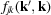depends not only on the wavevectors but also on the atomic environment, magnetic and orbital moments etc. From equation (1.11.4.1)and with the help of perturbation theory (Berestetskii et al., 1982), the atomic factorcan be expressed as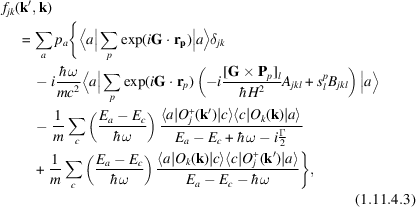where the first line describes the non-resonant Thomson scattering andis the energy width of the excited state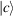. The second line gives non-resonant magnetic scattering with the spin and orbital terms given by the rank-3 tensors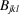(1.11.5.2)and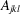(1.11.5.1), respectively. Compared to the second-to-last line, where the energy denominator can be close to zero, the last line is usually neglected, but sometimes it has to be added to the non-resonant terms, in particular at photon energies far from resonance. The third term gives the dispersion corrections also addressed as resonant scattering, magnetic and non-magnetic. In equation (1.11.4.3),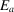andare the ground and excited states energies, respectively;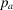is the probability that the incident state of the scatterer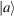is occupied; and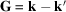is the scattering vector (in the case of diffraction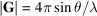, whereis the Bragg angle). The vector operator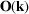has the form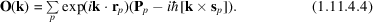The second term in this equation is small and is frequently omitted.

In general, the total atomic scattering factor looks like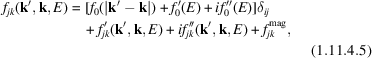whereis the ordinary Thomson (non-resonant) factor,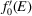and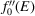are the isotropic corrections to the dispersion and absorption, which become stronger near absorption edges (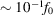), and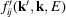and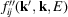are the real and imaginary contributions accounting for resonant anisotropic scattering and are sensitive to the local symmetry of the resonant atom and its magnetism. In the latter case, one should add the tensor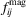(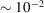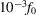) describing magnetic non-resonant scattering, which is also anisotropic (see the next section).

### References

Berestetskii, V. B., Lifshitz, E. M. & Pitaevskii, L. P. (1982). Quantum Electrodynamics. Oxford: Pergamon Press.Google Scholar
Bergevin, F. de & Brunel, M. (1972). Observation of magnetic superlattice peaks by X-ray diffraction on an antiferromagnetic NiO crystal. Phys. Lett. A, 39, 141–142.Google Scholar
Bergevin, F. de & Brunel, M. (1981). Diffraction of X-rays by magnetic materials. I. General formulae and measurements on ferro- and ferrimagnetic compounds. Acta Cryst. A37, 314–324.Google Scholar
Blume, M. (1985). Magnetic scattering of X-rays. J. Appl. Phys. 57, 3615–3618.Google Scholar
Blume, M. (1994). Resonant X-ray diffraction and polarization analysis at the iron K-edge. In Resonant Anomalous X-ray Scattering. Theory and Applications, edited by G. Materlik, C. J. Sparks & K. Fischer, pp. 91–97. Amsterdam: North-Holland.Google Scholar
Brunel, M. & de Bergevin, F. (1981). Diffraction of X-rays by magnetic materials. II. Measurements on antiferromagnetic Fe2O3. Acta Cryst. A37, 324–331.Google Scholar
Kolpakov, A. V., Bushuev, V. A. & Kuz'min, R. N. (1978). Dielectric permittivity in the X-ray region. Sov. Phys. Usp. 21, 959–977.Google Scholar
Platzman, P. M. & Tzoar, N. (1970). Magnetic scattering of X-rays from electrons in molecules and solids. Phys. Rev. B, 2, 3556–3559.Google Scholar